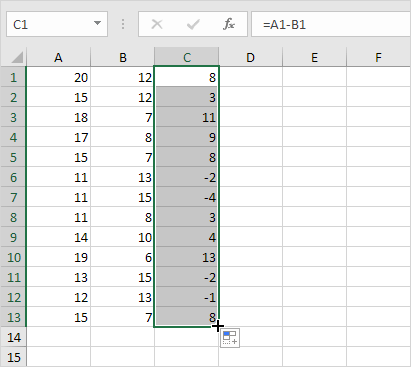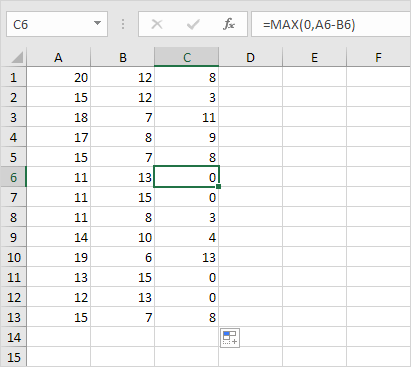# Negative Numbers to Zero

A clever Excel trick to change negative numbers to zero but leave positive numbers unchanged.

Below you can find two columns with numbers. To subtract the numbers in column B from the numbers in column A, execute the following steps.

1. Select cell C1.

2. Enter the formula =A1-B1

3. Click the lower right corner of cell C1 and drag the formula down.Result:4. To change the negative numbers to zero but leave the positive numbers unchanged, instead of =A1-B1, use =MAX(0,A1-B1) and drag the function down.

Result:Explanation: the MAX function returns the maximum of two values. If the result of the formula is negative, the function returns 0.